Test: Transfer Function - 2

# Test: Transfer Function - 2 - Electrical Engineering (EE)

Test Description

## 10 Questions MCQ Test GATE Electrical Engineering (EE) 2024 Mock Test Series - Test: Transfer Function - 2

Test: Transfer Function - 2 for Electrical Engineering (EE) 2023 is part of GATE Electrical Engineering (EE) 2024 Mock Test Series preparation. The Test: Transfer Function - 2 questions and answers have been prepared according to the Electrical Engineering (EE) exam syllabus.The Test: Transfer Function - 2 MCQs are made for Electrical Engineering (EE) 2023 Exam. Find important definitions, questions, notes, meanings, examples, exercises, MCQs and online tests for Test: Transfer Function - 2 below.
Solutions of Test: Transfer Function - 2 questions in English are available as part of our GATE Electrical Engineering (EE) 2024 Mock Test Series for Electrical Engineering (EE) & Test: Transfer Function - 2 solutions in Hindi for GATE Electrical Engineering (EE) 2024 Mock Test Series course. Download more important topics, notes, lectures and mock test series for Electrical Engineering (EE) Exam by signing up for free. Attempt Test: Transfer Function - 2 | 10 questions in 30 minutes | Mock test for Electrical Engineering (EE) preparation | Free important questions MCQ to study GATE Electrical Engineering (EE) 2024 Mock Test Series for Electrical Engineering (EE) Exam | Download free PDF with solutions
 1 Crore+ students have signed up on EduRev. Have you?
Test: Transfer Function - 2 - Question 1

### Non-minimum phase transfer function is defined as the transfer function

Detailed Solution for Test: Transfer Function - 2 - Question 1

The non-minimum phase transfer function is defined as the transfer function which has zeros (or) poles in right side of s-plane.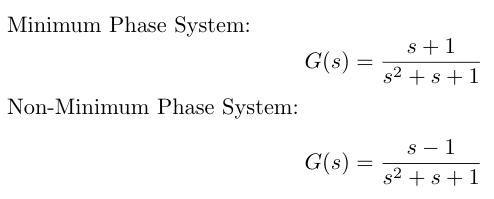Test: Transfer Function - 2 - Question 2

### The type and order of the system described by the open loop transfer function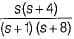are respectively

Detailed Solution for Test: Transfer Function - 2 - Question 2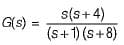Type of System: The Type of system denotes the no. of poles at the origin of the open loop transfer function G(s)H(s).

Hence, there is no open loop pole at origin. Hence, type of system = 0.

Order of a System: The order of a control system is determined by the highest power of 's' in the denominator of its transfer function.

Hence, highest power of s of the characteristic equation 1+ G(s) = 0 will be 2.
Hence, order of given System = 2.

Test: Transfer Function - 2 - Question 3

### Assertion (A): If the number of zeros are less than the number of poles (i.e. Z < P), we say that there are zeros at infinity and the order of such zeros is P-Z Reason (R): The value of the transfer function becomes zero for s tends to zero.

Detailed Solution for Test: Transfer Function - 2 - Question 3

For Z < P,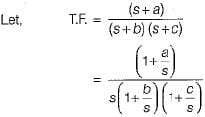∴ Here, number of zeros = 1 and no. of poles = 2
∴ P - Z = 1
When s →∞, transfer function becomes zero. Thus, there is one zero (P - Z = 1) at infinity. Thus, assertion is true.
Since value of transfer function becomes zero as s →∞ therefore, reason is false.

Test: Transfer Function - 2 - Question 4

The transfer function ofthe network shown below is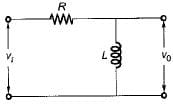Detailed Solution for Test: Transfer Function - 2 - Question 4

Let i be the current in the given circuit.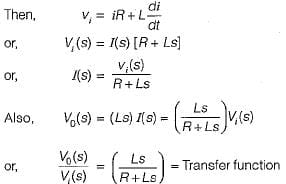Test: Transfer Function - 2 - Question 5

The principle of homogeneity and superposition are applied to

Detailed Solution for Test: Transfer Function - 2 - Question 5

Superposition theorem states that for two signals additivity and homogeneity property must be satisfied and that is applicable for the LTI systems.

Test: Transfer Function - 2 - Question 6

​The differential equation of a control system having input x(t) and output y(t) is given as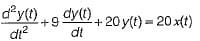The output response of the system for unit step input is given by

Detailed Solution for Test: Transfer Function - 2 - Question 6

Given differential equation is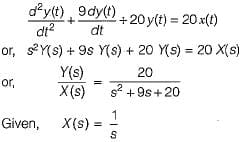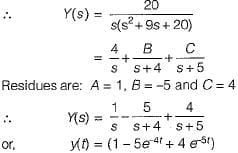Test: Transfer Function - 2 - Question 7

The step response of a system is given by
c(t) = 1 + 0.25 e-50t - 1.25e-10t
The steady state gain of the transfer function in time constant form will be

Detailed Solution for Test: Transfer Function - 2 - Question 7

Given, c(t) = 1 + 0.25 e-50t - 1.25e-10t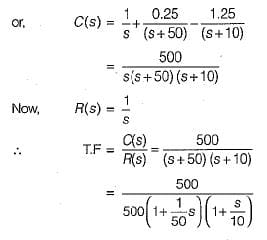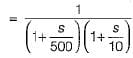Thus,C(s)/R(s) is in time constant form having steady state gain = 1.

Test: Transfer Function - 2 - Question 8

The poles and zeros of the transfer function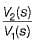for the network shown below are located at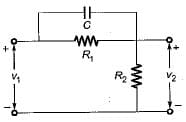Detailed Solution for Test: Transfer Function - 2 - Question 8

For given network, total input impedance is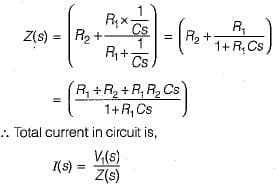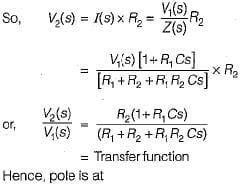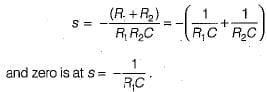Test: Transfer Function - 2 - Question 9

The pole-zero configuration of a transfer function is shown below: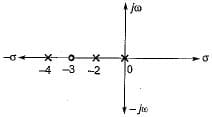If the value of the transfer function at s = 1 is 3.2, the gain factor K is

Detailed Solution for Test: Transfer Function - 2 - Question 9

From given pole-zero plot, transfer function is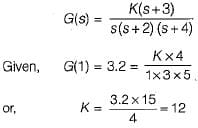So, gain factor K =12

Test: Transfer Function - 2 - Question 10

The type of a system denotes the number of

Detailed Solution for Test: Transfer Function - 2 - Question 10

The type of a system denotes the no. of poles at the origin of the open loop transfer function G(s)H(s).

## GATE Electrical Engineering (EE) 2024 Mock Test Series

23 docs|285 tests
Information about Test: Transfer Function - 2 Page
In this test you can find the Exam questions for Test: Transfer Function - 2 solved & explained in the simplest way possible. Besides giving Questions and answers for Test: Transfer Function - 2, EduRev gives you an ample number of Online tests for practice

## GATE Electrical Engineering (EE) 2024 Mock Test Series

23 docs|285 tests